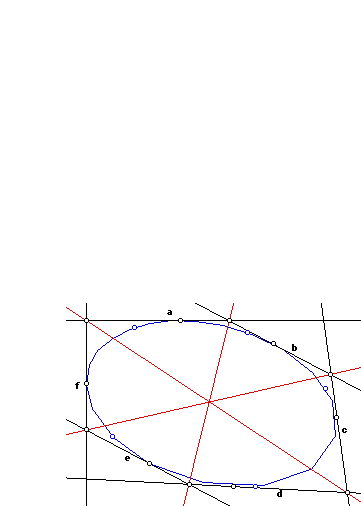Next: Some Stronger Theorems
Prev: Pascal's Theorem
Up: Projective Conics

# Brianchon's Theorem

Another way of stating Pascal's theorem is: If points ABCDEF lie on a conic, then points AB.DE, BC.EF, and CD.FA lie on one line. This shows the way to a dual theorem, known as Brianchon's theorem: if lines abcdef lie on a conic, then lines (a.b)(d.e), (b.c)(e.f), (c.d)(f.a) lie on one point. If six lines lie on a conic, then the hexagon which they form circumscribes the conic. So the more traditional form of Brianchon's theorem is:If a hexagon is circumscribed about a conic, its diagonals are concurrent.

Brianchon's theorem has a converse, too: If the diagonals of a hexagon are concurrent, then the hexagon may be circumscribed about a conic.

The proofs for these are exactly the duals of the proofs for Pascal's theorem and its converse.

Next: Some Stronger Theorems
Prev: Pascal's Theorem
Up: Projective ConicsThe Geometry Center Home Page